# ISEE Lower Level Math : How to find the decimal equivalent of a fraction

## Example Questions

← Previous 1 3 4 5 6 7 8 9

### Example Question #1 : How To Find The Decimal Equivalent Of A Fraction

In the number 1,063.8042, what place value is the 2 in?

thousandths

hundredths

ten thousandths

tenths

ones

ten thousandths

Explanation:

From left to right, let's begin with the numbers coming after (or to the right of) the decimal point.

.8042

8 is in the tenths place

0 is in the hundredths place

4 is in the thousandths place

2 is in the ten thousandths place

### Example Question #1 : How To Find The Decimal Equivalent Of A Fraction

What is 75% written as a fraction?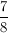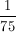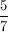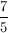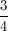Explanation:

Let's rewrite 75% as a fraction.

All percents are written out of 100.

So 75% is

75/100.

This fraction can be simplified.

If we divide both the numerator and denominator by 25,

75 ÷ 25 = 3

100 ÷ 25 = 4

Our simplified fraction will be written as### Example Question #1 : How To Find The Decimal Equivalent Of A Fraction

In the number, 24,977.68001, what place is the 8 in?

millionths

tenths

hundredths

ten thousandths

thousandths

hundredths

Explanation:

As you move away from the decimal point, the places grow from tenths on. Since the 8 is 2 places away from the decimal point, it is the hundredths.

### Example Question #2 : Fractions

Convert the fraction into a percent, rounded to the nearest whole number.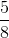13%

1.6%

63%

62%

16%

63%

Explanation:

Divide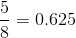Move the decimal two places to the right, 62.5%, then round up to the nearest whole number 63%.

### Example Question #3 : Fractions

Janie has a bag full of red, blue, orange, white, and green marbles. There are 5 of each color marble in the bag.

Janie removes all 5 green marbles and all 5 red marbles, then adds 2 blue, 2 orange and 9 white marbles. What percentage of blue marbles are there now?Explanation:

The adding and taking away leaves Janie with 7 blue marbles, 7 orange marbles and 14 white, and now 28 total marbles.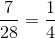or 25%.

### Example Question #1 : How To Find The Decimal Equivalent Of A Fraction

Which number is largest?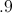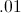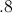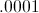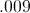Explanation:

Thinking about these numbers as fractions, we have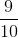,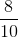,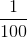,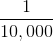, and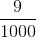.

The larger the number in the denominator is, the smaller the value of the total fraction. However, betweenand, the larger numerator is the bigger number. Therefore, the correct answer is .9.

### Example Question #1 : How To Find The Decimal Equivalent Of A Fraction

Showas a fraction.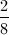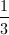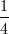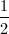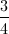Explanation:

First we rewriteas a fraction. Sinceis in the tenths place, we can write it over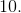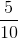This can be simplified by dividing the top and bottom number by.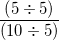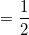### Example Question #1 : How To Find The Decimal Equivalent Of A Fraction

Write as a decimal: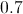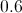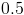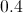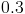Explanation: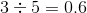, making this the decimal equivalent.

### Example Question #1 : How To Find The Decimal Equivalent Of A Fraction

What is the sum of 4.7 and 2.5?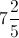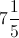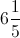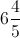Explanation:

4.7 and 2.5 add up to 7.2.

0.2 is equal to, so the correct answer is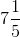.

### Example Question #8 : Fractions

Writeas a fraction.Explanation:

Rewrite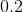as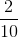.

Reduce the fraction:← Previous 1 3 4 5 6 7 8 9

### All ISEE Lower Level Math Resources